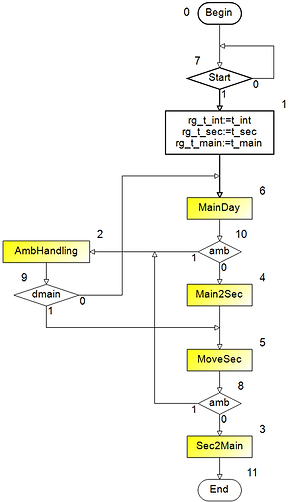top of page

Products | Synthagate—HLS & RTL | Examples of High Level and RTL Design | Internal Representation of ASMs

Internal Representation of ASMs

As you read above, designer can present ASM as a graph in ASM Creator or in System C or in VHDL. After that Synthagate numbers vertices, operators, microoperations and logical conditions and constructs two files – name.gsa and name.txt. Here name is the name of ASM. Such two files for ASM day (Fig. 1) are presented in Fig. 2 and Fig. 3. In Fig. 1 we have numbered vertices to explain Fig. 2 and Fig. 3.Figure 1. ASM day.asd with numbered vertices

File name.gsa is the two-connected list of ASM graph. Each row of this list corresponds to one vertex. Columns in this list:

1. The number of the vertex;

2. The content of the vertex – Yn for operator and xm for logical condition;

3. The number of the vertex following the operator vertex, or output ”1” of the conditional vertex;

4. The number of the vertex following output “0” of the conditional vertex.

Vertices Begin and End are described as operator vertices.Figure 2. ASM day.gsa

File name.txt (Fig. 3 for ASM day) contains three sections - Microinstructions (operators), Microoperations and Logical conditions.Figure 3. ASM day.txt

bottom of page